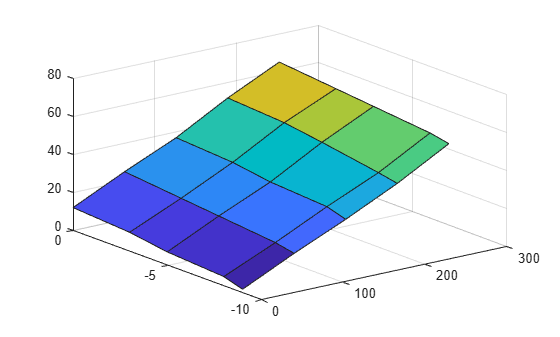# Working With ASAM CDFX Files in MATLAB

This example shows how to import a calibration data file into MATLAB, examine and modify its contents, and export the changes back to a file on disk.

### Importing a CDFX file

Import data from a CDFX file using the `cdfx` function.

`cdfxObj = cdfx("CDFXExampleFile.cdfx")`
```cdfxObj = CDFX with properties: Name: "CDFXExampleFile.cdfx" Path: "/tmp/Bdoc21a_1606923_197022/tpb1791c7c/vnt-ex38787800/CDFXExampleFile.cdfx" Version: "CDF20" ```

### Visualizing Calibration Data

CDFX files contain information about vehicle ECUs (systems), and their parameters (instances). Use `instanceList` and `systemList` to visualize the calibration data in table form. These functions also allow filtering based on instance or system short names.

`iList = instanceList(cdfxObj)`
```iList=4×6 table ShortName System Category Value Units FeatureReference ____________________________ _______________ __________ _________________ _______ _________________ "ASAM.C.SCALAR.GAIN" "ExampleSystem" "VALUE" {[ 3]} "gain" "FunctionScalar" "ASAM.C.SCALAR.BITMASK_0001" "ExampleSystem" "BOOLEAN" {[ 1]} "" "FunctionScalar" "ASAM.C.MAP" "ExampleSystem" "MAP" {1x1 struct } "" "Sample_Model_13" "ASAM.C.COM_AXIS" "ExampleSystem" "COM_AXIS" {[-9 -8 -5 -3 0]} "hours" "" ```

If you want to filter the table based on a desired short name, pass a string as a second argument.

`iListArray = instanceList(cdfxObj, "ASAM.C.SCALAR")`
```iListArray=2×6 table ShortName System Category Value Units FeatureReference ____________________________ _______________ _________ _____ ______ ________________ "ASAM.C.SCALAR.GAIN" "ExampleSystem" "VALUE" {} "gain" "FunctionScalar" "ASAM.C.SCALAR.BITMASK_0001" "ExampleSystem" "BOOLEAN" {} "" "FunctionScalar" ```

The default querying behavior will return a table for all instances whose short names partially match the search string. To filter for an exact instance name match, use the `ExactMatch` name-value pair.

`iListArrayExact = instanceList(cdfxObj, "ASAM.C.SCALAR.BITMASK_0001", "ExampleSystem", 'ExactMatch', true)`
```iListArrayExact=1×6 table ShortName System Category Value Units FeatureReference ____________________________ _______________ _________ _____ _____ ________________ "ASAM.C.SCALAR.BITMASK_0001" "ExampleSystem" "BOOLEAN" {} "" "FunctionScalar" ```

For CDFX files that contain calibration data for more than one ECU system, `systemList` can be useful to view the contents of each system at a high level.

`sList = systemList(cdfxObj)`
```sList=1×3 table ShortName Instances Metadata _______________ ____________ ________ "ExampleSystem" {1x4 string} "NO_VCD" ```

### Examining and Modifying Simple Calibration Parameters

Use `getValue` to extract the value of an instance from the CDFX object. Use `setValue` to modify the value of the instance.

`iValueScalar = getValue(cdfxObj, "ASAM.C.SCALAR.GAIN")`
```iValueScalar = 3 ```
```iValueScalarNew = iValueScalar + 20; setValue(cdfxObj, "ASAM.C.SCALAR.GAIN", iValueScalarNew); iValueScalarNew = getValue(cdfxObj, "ASAM.C.SCALAR.GAIN")```
```iValueScalarNew = 23 ```

### Working With More Complex Parameter Types

Certain instance categories contain more than just a physical value. These instances are often multi-dimensional arrays that are scaled according to an axis. Calling `getValue` on these instances returns a structure that contains each axis as a separate field, distinct from the PhysicalValue.

To inspect the CUBOID instance, first call `getValue`, then examine the properties of the returned structure. Notice that there is additional data associated with each axis, including the type of axis, its physical values, and whether the axis values are referenced from another instance on the CDFX object.

`iValueMap = getValue(cdfxObj, "ASAM.C.MAP")`
```iValueMap = struct with fields: PhysicalValue: [5x5 double] Axis1: [1x1 struct] Axis2: [1x1 struct] ```
`disp(iValueMap.PhysicalValue)`
``` 2 15 27 40 55 5 17 30 42 57 7 20 32 47 60 10 22 35 50 62 12 25 37 52 65 ```
`disp(iValueMap.Axis1)`
``` ReferenceName: "" Category: "STD_AXIS" PhysicalValue: [0 63 126 189 252] IsReferenced: 0 ```
`disp(iValueMap.Axis2)`
``` ReferenceName: "ASAM.C.COM_AXIS" Category: "COM_AXIS" PhysicalValue: [-9 -8 -5 -3 0] IsReferenced: 1 ```

We can also visualize the instance values using MATLAB plotting functions. For multidimensional arrays, use the physical values of the axes structures to define the axes on the plot.

```surf('ZDataSource', 'iValueMap.PhysicalValue', 'XDataSource', 'iValueMap.Axis1.PhysicalValue', 'YDataSource', 'iValueMap.Axis2.PhysicalValue') refreshdata;```Modifying the physical value of this instance works the same as for scalars. Update the physical value field of the structure and pass it back to `setValue`.

```iValueMap.PhysicalValue(:, 1) = iValueMap.PhysicalValue(:, 1)*2; setValue(cdfxObj, "ASAM.C.MAP", iValueMap);```

Now we can observe that the changes have been committed to the CDFX object in the workspace.

`iValueMapNew = getValue(cdfxObj, "ASAM.C.MAP")`
```iValueMapNew = struct with fields: PhysicalValue: [5x5 double] Axis1: [1x1 struct] Axis2: [1x1 struct] ```
`disp(iValueMapNew.PhysicalValue)`
``` 4 15 27 40 55 10 17 30 42 57 14 20 32 47 60 20 22 35 50 62 24 25 37 52 65 ```

To modify the axis values of this instance, we first need to know if the axis we want to modify is referenced or not. This can be determined by examining the `IsReferenced` field of each axis structure. If the axis is not referenced, we simply modify the `PhysicalValue` field of the axis structure and pass the top-level structure back to `setValue`.

`disp(iValueMapNew.Axis1.PhysicalValue)`
``` 0 63 126 189 252 ```
```iValueMapNew.Axis1.PhysicalValue = iValueMapNew.Axis1.PhysicalValue*10; setValue(cdfxObj, "ASAM.C.MAP", iValueMapNew); iValueMapNewAxis = getValue(cdfxObj, "ASAM.C.MAP"); disp(iValueMapNewAxis.Axis1.PhysicalValue)```
``` 0 630 1260 1890 2520 ```

However, some axes are not defined on the instance itself, and are instead referenced from another instance. There are specific instance categories for representing referenced axis values (COM_AXIS, RES_AXIS, and CURVE_AXIS). Attempting to modify a referenced axis from a referencing instance will result in an error. The solution is to update the values directly on the axis instance itself. Information on whether an axis is using referenced values, including the short name of the instance being referenced can be found on the axis fields of the top-level structure.

`iValueCommonAxis = getValue(cdfxObj, iValueMapNewAxis.Axis2.ReferenceName)`
```iValueCommonAxis = 1×5 -9 -8 -5 -3 0 ```
```iValueCommonAxis(:) = 1:5; setValue(cdfxObj, iValueMapNewAxis.Axis2.ReferenceName, iValueCommonAxis);```

Now that we have modified the original instance, we can observe that the changes are reflected in the referencing instance.

`iValueMapNew = getValue(cdfxObj, "ASAM.C.MAP")`
```iValueMapNew = struct with fields: PhysicalValue: [5x5 double] Axis1: [1x1 struct] Axis2: [1x1 struct] ```
`iValueMapNew.Axis2.PhysicalValue`
```ans = 1×5 1 2 3 4 5 ```

### Exporting Calibration Data to a File

You can write back to the same file, or to a new file by specifying a filepath.

`write(cdfxObj, "NewExampleFile.cdfx");`

## SupportGet trial now